# PHAML Software: problem classes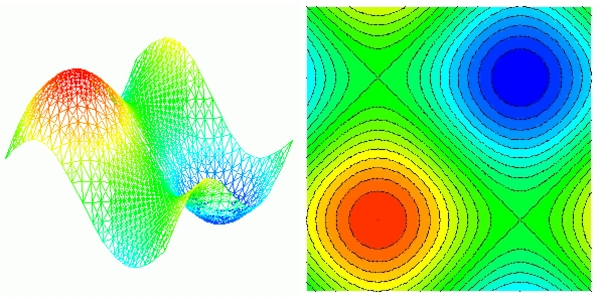Two visualizations of a solution computed by the PHAML software.

PHAML primarily solves linear, scalar, elliptic, partial differential equation boundary value problems in 2D, of the form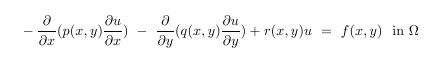where the domain is a bounded, connected, region in the plane. It can have curved boundaries defined by parametric equations (x(s),y(s)), and can have holes. The boundary conditions can be Dirichlet on part of the boundary,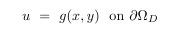and natural or mixed on the remainder of the boundary,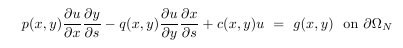Note that when p=q, or the boundary is parallel to the x or y axis, the natural boundary conditions reduce to Neumann boundary conditions.

Periodic boundary conditions are also supported.

PHAML also solves elliptic eigenvalue problems of the form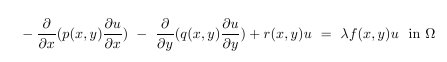where &lambda is an unknown eigenvalue. Multiple eigenvalues can be computed simultaneously, and they do not need to be at the ends of the spectrum. For eigenvalue problems, the boundary conditions must be homogeneous, i.e. g(x,y)=0.

PHAML also supports multicomponent solutions, or systems of elliptic PDEs, or vector PDEs. In this case, the solution u has k components, i.e. is a vector of length k, and there are k equations. In the above notation, p, q, and r are kXk matrices and f and g are vectors of length k.

PHAML provides for the retention and evaluation of an "old" solution to help in the solution of other classes of problems that are not supported directly. For example, parabolic PDEs can be solved by a one step method by using the old solution to evaluate the solution at the previous time step. Also, nonlinear PDEs can be solved by using the old solution to evaluate the previous solution in a nonlinear iteration. Algorithms that need more than one old solution (e.g. fourth order Runge-Kutta for time dependant problems) can often be formulated as a system of equations that PHAML can solve.

We are in the process of extending PHAML to solve 3D problems using bisected tetrahedra. This currently has very limited capability, but can solve some 3D problems. See the User's Guide for the current status.

Publications

Mitchell, W.F., PHAML User's Guide , NISTIR 7374 , 2006. (original, pdf, 3.2M ) (latest revision, pdf)